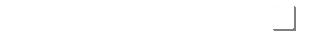# CALCULATOR / UNIT CONVERSION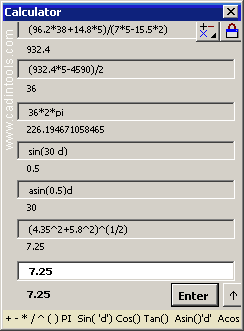•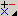/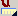: Calculator mode / Unit Conversion mode.
•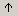Resize this window.

Note1: + addition, - subtract , * multiplication, / division, ( ) parenthesis, ^ exponent
Note2: PI = 3.14159265358979
Note3: Sin(), Cos(), Tan() : Trigonometric Functions.
Input angle in radians or degrees: sin(pi/2) = sin(90d) = 1.
Note4: Asin(), Acos(), Atan() :Inverse Trigonometric Functions.
Output angle in radians or degrees: asin(1) = pi/2, asin(1)d = 90º.
Note5: Square root= <value>^(1/2), Cube root = <value>^(1/3).
Note6: "." or "," are accepted as decimal point (it depends of the Windows Regional Configuration).
Note7: You can use formulas in any other window (Scale, Curve Edition, Transformation, ...)